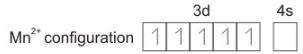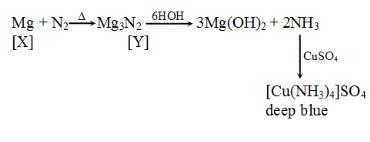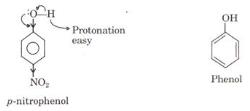Courses

# Sample BITSAT Chemistry Test

## 40 Questions MCQ Test BITSAT Mock Tests Series & Past Year Papers | Sample BITSAT Chemistry Test

Description
This mock test of Sample BITSAT Chemistry Test for JEE helps you for every JEE entrance exam. This contains 40 Multiple Choice Questions for JEE Sample BITSAT Chemistry Test (mcq) to study with solutions a complete question bank. The solved questions answers in this Sample BITSAT Chemistry Test quiz give you a good mix of easy questions and tough questions. JEE students definitely take this Sample BITSAT Chemistry Test exercise for a better result in the exam. You can find other Sample BITSAT Chemistry Test extra questions, long questions & short questions for JEE on EduRev as well by searching above.
QUESTION: 1

### Hydrogen bonding is maximum in

Solution:
ethyl chloride & triethyl doesnt have hydrogen bonding because of absence of N/O/F group.

di ethyl ether also doesnt have h-bonding because the h atom is not bonded with Oxygen.

ethanol has h-bonding because the H atom is joint with O atom & hence it has the maximum.
QUESTION: 2

Solution:
QUESTION: 3

### Which of the following does not have sp2-hybridised carbon?

Solution:
QUESTION: 4

The total number of electrons present in all the s-orbitals, all the p-orbitals and all the d-orbitals of cesium ion are respedtively

Solution:
Cs+ = 2,8, 8,18,18
No. of 's' electrons = 2 + 2 + 2 + 2 + 2 = 10
No. of 'p' electrons = 6 + 6 + 6 + 6 = 24
No. of 'd' electrons = 10 + 10 = 20
QUESTION: 5

The effect of atoms or groups losing electrons towards a carbon atom is called

Solution:
QUESTION: 6

The number of significant figures in 40.00 is

Solution:
QUESTION: 7

The weight of one molecule of a compound C₆₀H₁₂₂ is

Solution:

The Molecule iS C₆₀H₁₂₂
Molecular mass of C₆₀H₁₂₂ --- [60x12+1x122]
=720+122
=842 Unified mass
Weight of one molecule of C₆₀H₁₂₂ :
=molecular weight of C₆₀H₁₂₂ / Avogadro's number
=842/6.023x10²³
=1.4x10⁻²¹ g

∴The weight of a molecule of a compound C₆₀H₁₂₂ is 1.4x10⁻²¹ g

QUESTION: 8

Which one of the following statements is true for proteins?

Solution:
Function of Proteins:
(i) Proteins occur as food reserves as glutelin, globulin casein in milk.

(ii) They act as antibodies and as hormones.

(iii) Proteins are the most diverse molecule on the earth.

(iv) Proteins work as hormone as insulin and glucagon.

(v) Antibiotics as gramicidin, tyrocidin and penicillin are peptides.

(vi) They are structural component of cell.

(vii) They are biological buffers.

(viii) Proteins helps in defence, movement activity of muscles, visual pigments receptor molecules, etc.

QUESTION: 9

The no. of unpaired electrons in Mn2+ is

Solution:QUESTION: 10

The alcohol obtained by the hydrolysis of oils and fats is

Solution:
QUESTION: 11

Which of the following is paramagnetic species?

Solution: Potassium superoxide is a yellow paramagnetic solid that is air and moisture sensitive. Its formula is KO2 and because potassium is +1, the superoxide has a charge of -1. ... The unpaired electron in the pi* orbital accounts for the paramagnetic behavior or potassium superoxide.
QUESTION: 12

The heat of neutralisation is maximum when

Solution:
QUESTION: 13

Reaction of acids with alcohols is also known as

Solution:
QUESTION: 14

In a reversible chemical reaction having two reactants in equilibrium, if the concentration of the reactants are doubled then the equilibrium constant will

Solution:
QUESTION: 15

Which one of the following is an incorrect statement?

Solution:

The electron affinity of fluorine is unexpectedly less than that of Cl. Because the size of F atom is quite small, as a result, the electron-electron repulsions in the relatively compact 2p-subshell are comparitively large and hence the incoming electron is not accepted with the same ease as in the case with Cl.

QUESTION: 16

The rate of a gaseous reaction is given by the expression K [A][B]. If the volume of the reaction vessel is suddenly reduced to 1/4th of the initial volume, the reaction rate relating to original rate will be

Solution:
Rate = kab. When volume is reduced to 1/4th, concentrations will become 4 times.
New rate=k(4a)(4b)=16kab=16 times.
QUESTION: 17

The no. of unpaired electrons in the complex ion [CoF₆]3⁻ is (Atomic no. Co = 27)

Solution:
QUESTION: 18

The law formulated by Nernst is

Solution:
The third law was developed by the chemist Walther Nernst during the years 1906-1912. It is often referred to as Nernst’s theorem or Nernst’s postulate. Nernst proposed that the entropy of a system at absolute zero would be a well-defined constant. Instead of being 0, entropy at absolute zero could be a nonzero constant, due to the fact that a system may have degeneracy (having several ground states at the same energy).
QUESTION: 19

2, 4, 6-Trinitrophenol is a/an

Solution:
An acid is a compound which can donate the protons easily.Carboxylic acids are termed as acids because they follow the definition of acid.So, the presence of -COOH group is not the criteria for being an acid.

In case of Picric acid, the phenolic -OH group readily give away proton as the resulting phenoxide ion is significantly stabilized by delocalization over  three nitro groups situated at 2-,4-,6- positions.So it is also an acid.
QUESTION: 20

The group of elements which are not transition elements but are discussed with them is

Solution:
QUESTION: 21

Which of the following is formed when K₂Cr₂O₇ reacts with concentrated H₂SO₄ in cold?

Solution:
QUESTION: 22

A depolariser used in dry cell is

Solution: A common dry cell is the zinc-carbon cell, sometimes called the dry Leclanche cell, with a nominal voltage of 1.5 volts, the same as the alkaline cell (since both use the same zinc–manganese dioxide combination).
QUESTION: 23

Marsh gas contains which of the following

Solution:
QUESTION: 24

Which one of the following is explosive and readily hydrolysed?

Solution:
QUESTION: 25

The first product obtained during fractional distillation of petroleum is

Solution:
QUESTION: 26

Which of the following is a strong electrolyte?

Solution:
QUESTION: 27

In the keto-enol tautomerism of dicarbonyl compounds the enol form is preferred in contrast to the keto form, this is due to

Solution:
QUESTION: 28

Chlorobenzene on heating with aq NH₃ under pressure in the presence of cuprous chloride gives

Solution: Aniline is an organic compound with the formula C6H5NH2. Consisting of a phenyl group attached to an amino group, aniline is the prototypical aromatic amine. Its main use is in the manufacture of precursors to polyurethane and other industrial chemicals. Like most volatile amines, it has the odor of rotten fish.
QUESTION: 29

BF₃ acts as an acid according to the concept of

Solution: It can accept a lone pair of electrons. So it acts as Lewis acid.
QUESTION: 30

Nitrogen is relatively inactive element because

Solution:
Nitrogen is relatively inactive element because bond dissociation energy of its molecule is fairly high.
N≡N bond energy is very high 945kJmol^−1.
QUESTION: 31

A metal X on heating in nitrogen gas gives Y, Y on treatment with H₂O gives a colourless gas which when passed through CuSO₄ soution gives a blue colour. Y is

Solution:QUESTION: 32

In diborane, the two H - B - H angles are nearly

Solution:
QUESTION: 33

One of the oxidants used with liquid propellants is

Solution:
QUESTION: 34

What are the products formed when an aqueous solution of magnesium bicarbonate is boiled

Solution:
QUESTION: 35

Sodium is made by the electrolysis of a molten mixture of about 40% NaCl and 60% CaCl₂ because

Solution: Its melting point is so high and it can can melt under drastic condition. So this mixture is used to lower its melting point.
QUESTION: 36

Benzene and toluene form nearly ideal solutions. At 20oC, vapour pressure of benzene is 75 torr and that of toluene is 22 torr. The partial vapour pressure of benzene at 20oC for a solution containing 78 g of benzene and 46 g of toluene is ___ torr.

Solution: Since benzene and toulene form nearly ideal solutions, we can apply raoult`s law
that is the partial pressure of benzene= mole fraction * vapour pressure of benzene in pure form
=2/3*75
=50
QUESTION: 37

The turbidity of a polymer solution measures

Solution:
QUESTION: 38

Which of the following has Frenkel defects?

Solution:
QUESTION: 39

X litres of CO is present at STP. It is completely oxidised to CO2. The volume ofCO2 formed is 11.2 litres at STP. What is the value of X.

Solution:
The balanced cheical rection is:
CO      +     1/2O2                -------------->            CO2
moles = 11.207/22.4 = > 1.9735
moles of CO = is also, 1.9735 from mole – 1 analysis.
Hence volume at STP for CO is:
1.9735  = X/22.4
that is same  as  = > 11.207 litre.
Hence the same Volume will be oxidized.
QUESTION: 40

The adsorption of solids, from a solution, is called

Solution: When a solute(here solid ) is adsorbed from a solution is called Positive adsorption vice versa when a solvent is adsorbed it is called negative adsorption.

Track your progress, build streaks, highlight & save important lessons and more!

### Similar Content### Related tests